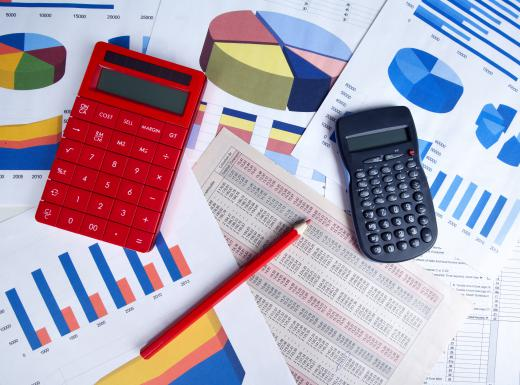# What is a Frequency Table?

Darlene Goodman
Darlene GoodmanThe data from frequency tables may be made into pie charts to show the values in a more visually appealing way.

A frequency table is an organized count of items or values. In its simplest form, a frequency table shows how frequently each particular value occurs in a data set. The raw data is organized into classes and counted to show how many of each class exists in the raw data. These tables are often useful for discovering basic statistical information about a data set.

If there are only a few values in the raw data set, a simple frequency table is usually sufficient. Some data sets are too large or unorganized to work a table by hand, and the assistance of a calculator or computer may be necessary. Generally, simple frequency tables consist of three columns, used to track categories known as mark, tally, and frequency.

The mark column usually lists all the possible classes in the data set. For example, if someone flipped a coin 30 times, he or she would have 30 values in the data set, but only two classes — heads and tails. On the other hand, if three people got dessert from a shop with 25 different ice cream flavors, there would be three values in the data set and 25 possible classes.

With larger data sets, the process of creating a frequency table typically requires that raw data be organized into groups or ranges, called class intervals. This can be useful when dealing with number values. For example, if the lowest number of a data set is one, and the highest number is 300, the mark column could be broken into six class intervals, starting with one-50, 51-100, 101-150, and so on.

Next, the organized data is commonly counted to determine the number of times each class occurs in the data set. In a simple frequency table, this is usually accomplished by hand marking the tally column, next to the class row, once for each value, or count. In a more complex data set, the raw data is often entered into a computer or calculator instead of being hand tallied.

The third column in a frequency table is the frequency column. This is where the frequency of occurrences for each class is listed. The numbers in this column are not the raw data values, but rather the number of times each class occurred in the data set. This column is often made into a graph or a pie chart to show the values in a more visually interesting way.

Frequency tables can be useful to uncover some basic statistical data about a raw data set. They may show any modes, or the values that occur most often, in the data. These tables may also be helpful for locating the median, or middle value, because the data is often organized in order of magnitude in the mark column.

A frequency table may also be used to determine the distribution of the data. These are often called frequency distribution tables for this reason. A table may also show whether the data has a central tendency, which can be visually represented by higher frequencies clustered in the center of the table. It may also display the dispersion of the data over all classes, which is also called its variability.